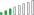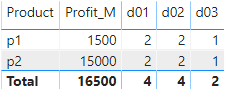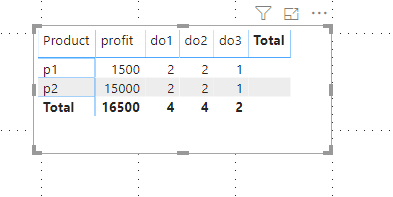cancel
Showing results for
Did you mean:Helper I

Hi

I am new to powerbi , I wanted to add additional column(Profit) in the matrix . Please refer the table below.

Can the expected below is possible in Matrix ?

Table

 Customeid Product Decile Profit 1 p1 d01 100 2 p1 d01 200 3 p1 d02 300 4 p1 d02 400 5 p1 d03 500 11 p2 d01 1000 12 p2 d01 2000 13 p2 d02 3000 14 p2 d02 4000 15 p2 d03 5000 Expected product profit do1 do2 do3 p1 1500 2 2 1 p2 15000 2 2 1
5 REPLIES 5Resolver III

@Gchandrukris , you can also break down your mesures into small little components this way:

Profits = SUM('Table'[Profit])

Decile Count = COUNT('Table'[Decile])
d0 1 = CALCULATE([Decile Count],'Table'[Decile] = "d01")
d0 2 = CALCULATE([Decile Count],'Table'[Decile] = "d02")
d0 3 = CALCULATE([Decile Count],'Table'[Decile] = "d03")

You can then add Product Field and the Measures Profits, d01, d02 and d03 to your Matrix.

If this solution works for you, kindly kudo my response and mark as solution to allow others benefit from it.Helper I

Hi,

As you mentioned, I have created a separate measures for each column which works fine.

In my case my measures calculates sales / sum(sales) for all columns. I wanted to show in the tooltip sales & totalsales.

eg

category  Measure1  Measure2 Measure3

A              10             20             30

B              20             30             30

after calculating the percentage

category  Measure1  Measure2 Measure3

A             17 %           33%          50%

B              25%            37.5%          37.5%

In my tool on 17% I wanted to show s

Sales: 10

TotalSales : 60

can you help me on thisAnonymous
Not applicable

Hello!!

First create this measures

``````Profit_M = sum(Hoja5[Profit])

d01 = CALCULATE(COUNT(Hoja5[Product]);Hoja5[Decile] = "d01")

d02 = CALCULATE(COUNT(Hoja5[Product]);Hoja5[Decile] = "d02")

d03 = CALCULATE(COUNT(Hoja5[Product]);Hoja5[Decile] = "d03")``````

Finally, put all together in a matrix.Regards!!Helper I

Thanks for your response. Is there any other way other than creating measures. Because Deciles are dynamic. it keeps increasing.Community Support

Create a new table,set"profit","d01","d2","d03" as the row value of the column:Then create a measure as below:

``````Measure =
SWITCH(SELECTEDVALUE('Table (2)'[Column]),"profit",SUM('Table'[Profit]),"do1",CALCULATE(COUNT('Table'[Product]),'Table'[Decile]= "d01"),"do2",CALCULATE(COUNT('Table'[Product]),'Table'[Decile]= "d02"),
"do3",CALCULATE(COUNT('Table'[Product]),'Table'[Decile]= "d03"),BLANK())``````

Finally you will see:In my suggestion,only one measure is needed ,I'm not sure whether it is what you need?

Best Regards,
Kelly